# 用 JavaScript 學習資料結構和演算法：字典（Dictionary）和雜湊表（Hash Table）篇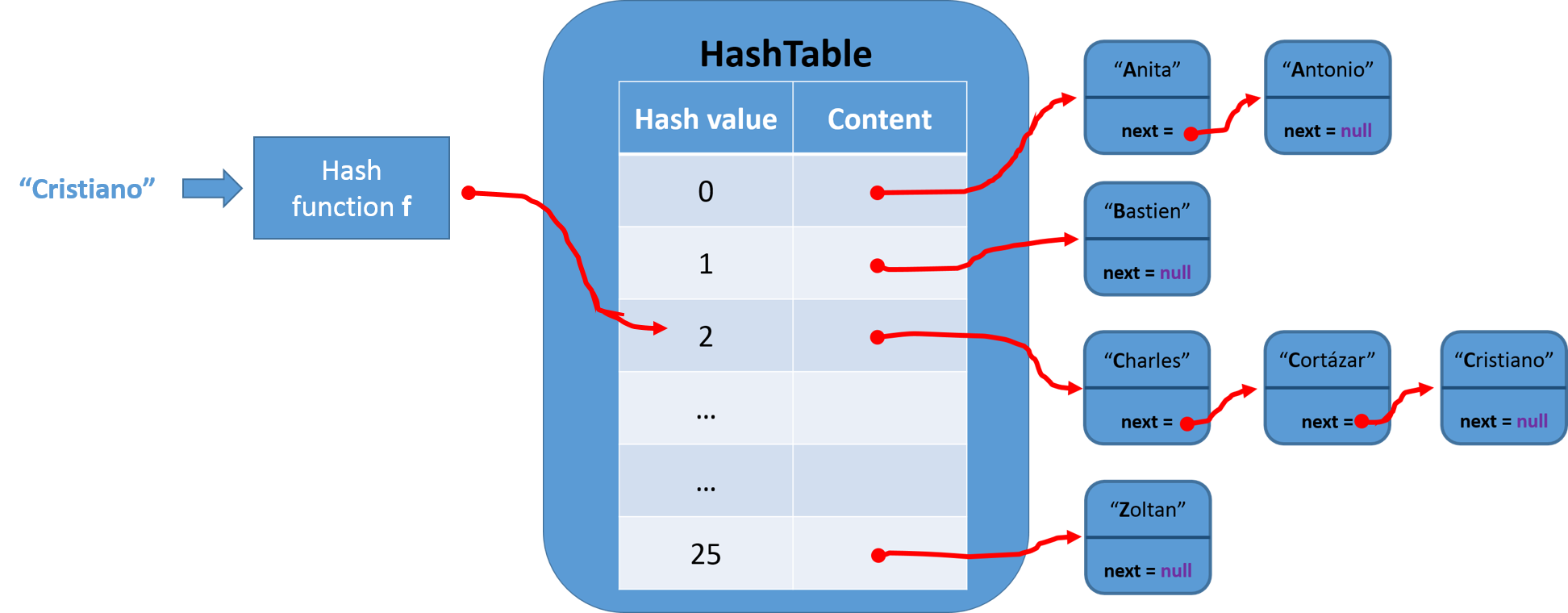# 什麼是字典（Dictionary）？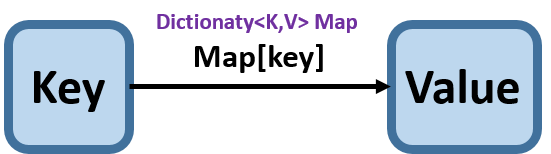1. 建立字典類別
與 Set 的情況類似，在 ES6 中也有原生的 Map 類別實作，因此本章我們也參考 ES6 的 Map 類別進行實作：

function Dictionary() {
let items = {};
this.set = function(key, value) {};
this.remove = function(key) {};
this.has = function(key) {};
this.get = function(key) {};
this.clear = function() {};
this.size = function() {};
this.keys = function() {};
this.values = function() {};
}

• has(key)：判斷是 key 是否存在，這在 Dictionary 尤其重要
this.has = function(key) {
return key in items;
// 也可以使用 return items.hasOwnProperty(key);
}

• set(key, value)：新增一個鍵值
this.set = function(key, value) {
items[key] = value;
};

• remove()：刪除一個鍵值
this.remove = function() {
if(this.has(key)) {
delete items[key];
return true;
}
return false;
};

• get()：讀取一個值
this.get = function() {
// 先判斷是否存在鍵
return this.has(key) ? items[key] : undefinded;
};

• values()：回傳所有值
this.values = function() {
let values = {};
for(let k in items) {
if(this.has(k)) {
values.push(items[k]);
}
}
return values;
};

• clear()：清除字典
this.clear = function() {
items = {};
};

• size()：回傳字典長度
1. 使用類似於 Linked List 的 length 變數計算法，當新增、刪除元素時順便增減長度
this.size = function() {
return length;
}

1. 使用 JavaScript 內建 Object 類別的內建函數 keys
this.size = function() {
return Object.keys(items).length;
}

1. 手動迭代判斷是否存在集合中並累加個數
this.size = function() {
let count = 0;
// 特別注意在 JavaScript 中 for in 會一起把繼承於 Object 類別和物件自身的所有相關非相關資料結構的屬性一起迭代出來
for(let prop in items) {
// 判斷是否屬於 items 的屬性
if(items.hasOwnProperty(prop)) {
++count;
}
return count;
}
}

• keys()：回傳字典擁有的鍵
this.keys = function() {
return Object.keys(items);
}

• getItems()：回傳字典
this.getItems() {
return items;
};

2. 使用字典

const dictionary = new Dictionary();
// 新增鍵值
dictionary.set('Mark', 'mark@gmail.com');
dictionary.set('Ivy', 'ivy@gmail.com');
dictionary.set('Mary', 'mary@gmail.com');

console.log(dictionary.keys());
console.log(dictionary.values());
console.log(dictionary.get('Mark'));
dictionary.remove('Mark');
console.log(dictionary.keys());


# 什麼是雜湊表（Hash Table）？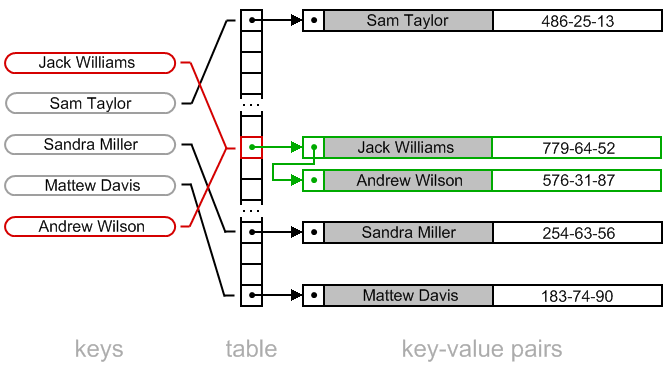1. 建立雜湊表

function HashTable() {
let table = [];
let getHashTableCode = function(key) {
let hash = 0;
for(let i = 0; i < key.length; i++) {
// charCodeAt 會回傳指定字串內字元的 Unicode 編碼（可以包含中文字）
hash += key.charCodeAt(i);
}
// 為了取到較小值，使用任意數做除法 mod 處理
return hash % 37;
}
this.put = function(key, value) {
// 根據 key 取出 Hash Function 產生的 position
const position = getHashTableCode(key);
// 對應 key 和 value
table[position] = value;
}
this.get = function(key) {
// 根據 key 取出 Hash Function 產生的 position
const position = getHashTableCode(key);
// 回傳值
return table[position];
}
this.remove = function(key) {
// 根據 key 取出 Hash Function 產生的 position
const position = getHashTableCode(key);
// 由於我們不能改變陣列長度（因為會影響到其它對應位置），所以將值給定 undefinded
table[position] = undefinded;
}
}

2. 使用雜湊表

let hashTable = new HashTable();
hashTable.put('Mark', 'mark@gmail.com');
hashTable.put('Ivy', 'ivy@gmail.com');
hashTable.put('Mary', 'mary@gmail.com');

hashTable.get('Mark');
hashTable.get('Jack');
// 刪除鍵值為 Mark 資料
hashTable.remove('Mark');
hashTable.get('Mark');

3. 處理雜湊表衝突

在上面實作的過程中，聰明的讀者應該可以發現：雖然雜湊表方便好用，但有可能會有不同鍵值但有相同雜湊值的情況出現（例如：Jamie 和 Sue 的值 ASCII Code 相加就相同）。這種不同值卻在雜湊表對應相同位置的情況我們稱作衝突（collision），若不處理的話，後來的值會覆蓋掉之前的值。接下來我們會介紹三種方式來避免碰撞（collision）。

• 分離鏈結：分離鏈結是在每一個 hash value 放置一個鏈結串列，當有 hash key 相同的值要放入時就新增進鏈結串列，是一個相對簡單的方式，但需要額外的鏈結串列空間。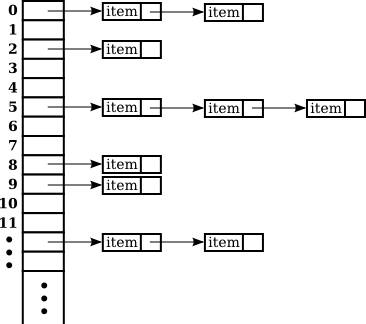更新過後的 HashTable：

function LinkedList() {
const Node = function(element) {
this.element = element;
this.next = null;
}
let length = 0;
// 第一個節點的指標
// 在尾部新增一個節點
this.append = function(element) {};
// 在特定位置新增一個元素節點
this.insert = function(position, element) {};
// 從串列中移除一個元素節點
this.remove = function(element) {};
// 從串列中移除一個特定的節點
this.removeAt = function(position) {};
// 回傳元素在串列的元素節點 index，若無則回傳 -1
this.indexOf = function(element) {};
// 判斷串列是否為空，是回傳 true，反之 false
this.isEmpty = function() {};
// 回傳串列元素個數
this.size = function() {};
// 由於 Node 是一個物件，所以運用 toString 方法將資料值輸出
this.toString = function() {};
// 列印出值
this.print = function() {};
}

function HashTable() {
let table = [];
// 實作內部一個 ValuePair 類別，存原始 key、value
let ValuePair = function(key, value) {
this.key = key;
this.value = value;
}
let getHashTableCode = function(key) {
let hash = 0;
for(let i = 0; i < key.length; i++) {
// charCodeAt 會回傳指定字串內字元的 Unicode 編碼（可以包含中文字）
hash += key.charCodeAt(i);
}
// 為了取到較小值，使用任意數做除法 mod 處理
return hash % 37;
}
this.put = function(key, value) {
let position = getHashTableCode(key);
// 若該位置沒有新增過元素，要先 init 一個 LinkedList 類別
if(table[position] === undefinded) {
}
table[position].append(new ValuePair(key, value));
}
this.get = function(key) {
let position = getHashTableCode(key);
if(table[position] !== undefinded) {
// 使用迴圈尋找在 LinkedList 中符合 key 的元素
while(current.next) {
if(current.element.key === key) {
return current.element.value;
}
current = current.next;
}
// 若是要找的是第一個或是最後一個節點的情況就不會進入 while 迴圈，要在這裡處理
if(current.element.key === key) {
return current.element.value;
}
}
return undefinded;
}
// 與一般雜湊表不同，我們要移除的是鏈結串列的元素
this.remove = function(key) {
let position = getHashTableCode(key);
// 判斷該位置是否有元素
if(table[position] !== undefinded) {
while(current.next) {
// 當 key 相同時使用 LinkedList 的 remove 移除元素
if(current.element.key === key) {
table[position].remove(current.element);
if(table[position].isEmpty()) {
table[position] = undefinded;
}
// 若成功移除則回傳 true
return true;
}
}
current = current.next;
}
// 若是要找的是第一個或是最後一個節點的情況就不會進入 while 迴圈，要在這裡處理
if(current.element.key === key) {
table[position].remove(current.element);
if(table[position].isEmpty()) {
table[position] = undefinded;
}
}
}
return false;
}

• 線性探查：是另外一種解決雜湊表，若是欲加入 Hash Table 位置已經被佔據則往下一個 index + 1 去填，若還是被佔據則考慮 index + 2，以此類推。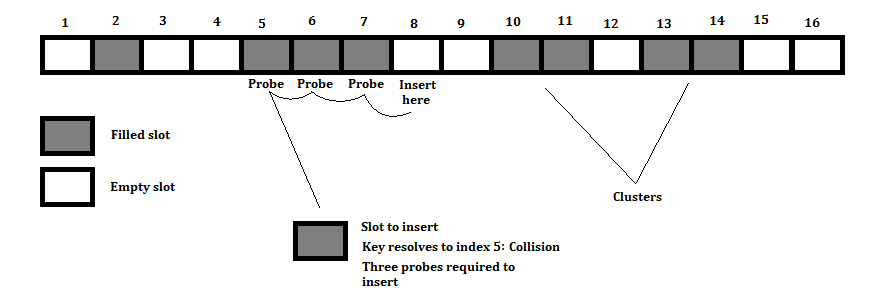更新過後的 HashTable：

function HashTable() {
let table = [];
// 實作內部一個 ValuePair 類別，存原始 key、value
let ValuePair = function(key, value) {
this.key = key;
this.value = value;
}
let getHashTableCode = function(key) {
let hash = 0;
for(let i = 0; i < key.length; i++) {
// charCodeAt 會回傳指定字串內字元的 Unicode 編碼（可以包含中文字）
hash += key.charCodeAt(i);
}
// 為了取到較小值，使用任意數做除法 mod 處理
return hash % 37;
}
// 由於 JavaScript 陣列可動態增加長度，所以不用擔心長度不夠問題
this.put = function(key, value) {
let position = getHashTableCode(key);
// 若是位置沒被佔據直接 new 一個 ValuePair，若有則考慮下一個 index
if(table[position] === undefinded) {
table[position] = new ValuePair(key, value);
} else {
let index = ++position;
while(table[index] !== undefinded) {
index++;
}
table[index] = new ValuePair(key, value);
}
}
this.get = function(key) {
let position = getHashTableCode(key);
// 先確認鍵值是否存在
if(table[position] !== undefinded) {
// 開始比對，沒有就下一個
if(table[position].key === key) {
return table[position].value;
} else {
let index = ++position;
while(table[index] === undefinded || table[index].key !== key) {
index++;
}
if(table[index].key === key) {
return table[index].value;
}
}
}
return undefinded;
}
this.remove = function(key) {
let position = getHashTableCode(key);
// 先確認鍵值是否存在
if(table[position] !== undefinded) {
// 開始比對，沒有就下一個
if(table[position].key === key) {
table[index] = undefinded;
} else {
let index = ++position;
while(table[index] === undefinded || table[index].key !== key) {
index++;
}
if(table[index].key === key) {
table[index] = undefinded;
}
}
}
return undefinded;
}
}

• 更好的雜湊表（Hash Function）：俗話說預防勝於治療，若是能使用更好的 Hash Function 就能預先避免衝突（collision）的可能（擁有較低插入和檢索元素的時間、較低衝突的可能意味著更好的雜湊表）。
// djb2HashCode 實作
let djb2HashCode = function(key) {
// 初始化 hash 值，大部分實作使用 5381
let hash = 5381;
for(let i = 0; i < key.length; i++) {
// 根據經驗值給個魔術數字 33
hash = hash * 33 + key.charCodeAt(i);
}
// 1013 為隨機質數
return hash % 1013;
}


# 總結

1. 什麼是字典（Dictionary）？如何使用？
2. 什麼是雜湊表（Hash Table）？如何實作？
3. 處理雜湊表衝突

# 延伸閱讀

（image via algolistmcgillmindcrackerinchwscecilsunkure

@kdchang 文藝型開發者，夢想是做出人們想用的產品和辦一所心目中理想的學校。A Starter & Maker. JavaScript, Python & Arduino/Android lover.:)